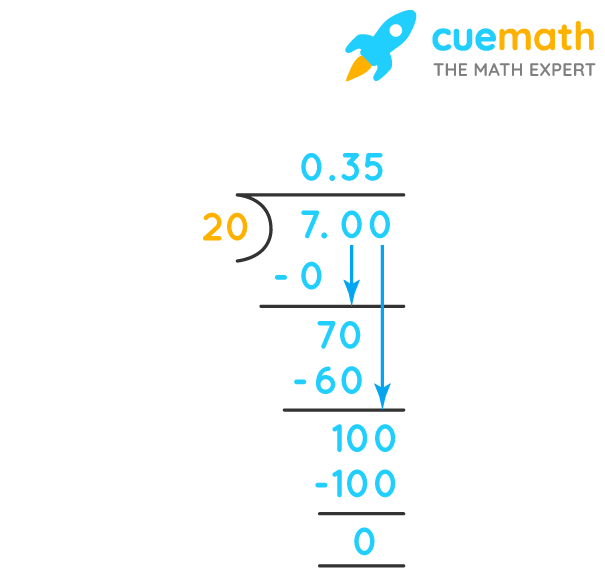# What Is 7 20 as a Decimal

## Question: What is 7/20 as a decimal?

The fractions and decimals are just two ways to represent the same numbers.

## Answer: 7/20 as a decimal is equal to 0.35.

Let's convert the given fraction to a decimal.

## Explanation:

To convert any fraction to decimal form, we just need to divide its numerator by denominator.

Here the fraction is 7/20 which means we need to perform 7 ÷ 20.### Alternate Method:

It is difficult to perform a division when a dividend is less than the divisor.

So, we re-write 7/20 as (7/20) × 100/100.

So, (7/20) × 100 = 7 × 5 = 35

Now, 35 ÷ 100 = 0.35

Therefore, 7/20 = (7/20) × 100/100 = 35/100 = 0.35